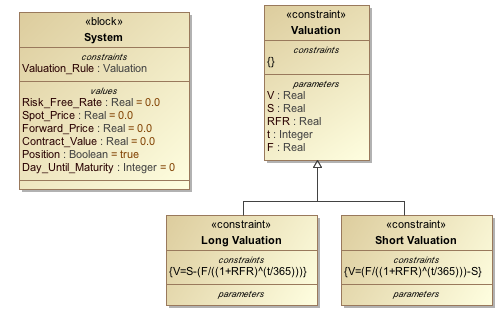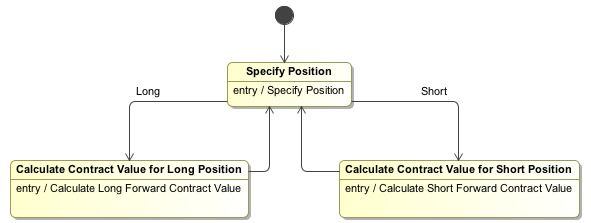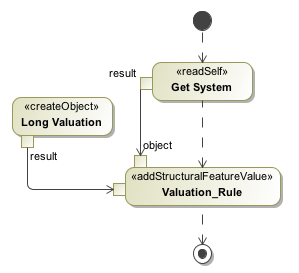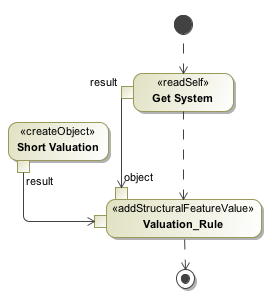Cameo Simulation Toolkit 19.0 LTR Documentation

The concept of dynamic constraint is to allow parametric calculation of a Constraint Property with another constraint rather than one from its type (Constraint Block). Such constraint can be dynamically obtained during the simulation from typing Constraint Block or one of its subtypes. This section uses the ForwardContractValuation.mdzip sample to demonstrate this concept.

To dynamically apply different constraints to a Constraint Property

1. Create a Constraint Block with all needed Constraint Parameter(s).
2. Create Constraint Blocks which inherit the Constraint Block created in step 1.
3. Specify the constraint expression of each Constraint Blocks created in step 2.
4. Type the Constraint Property with the Constraint Block created in step 1.
5. Create a behavior, for example State Machine, that will assign the runtime object of different Constraint Blocks created in step 2 to the Constraint Property.

In ForwardContractValuation.mdzip, we will dynamically assign a constraint to the Valuation_Rule Constraint Property, which is typed by the Valuation Constraint Block (having two subtypes: Long Valuation and Short Valuation Constraint Blocks with two different constraint expressions) as shown in the following figure. The context of this simulation will be the System Block.

######A Valuation Constraint Block as the Type of the Valuation_Rule Constraint Property.

In the following figure, the state machine is used as the classifier behavior of the System block. The Calculate Contract Value for Long Position and Calculate Contract Value for Short Position states apply different valuations to the System block via its entry activity.

######System Classifier Behavior State Machine.

The Calculate Contract Value for Long Position's entry activity assigns the runtime value of the Long Valuation Constraint Block to the Valuation_Rule Constraint Property (see the first figure below). Consequently, the values of the constraint parameters will be calculated with the constraint expression specified in Long Valuation

######Entry Activity of Calculate Contract Value for Long Position State.

Similarly, the second figure below demonstrates the Short Valuation dynamic assignment.## Sample model

The model used in the figures on this page is the ForwardContractValuation.mdzip sample model that comes with the modeling tool.

To open this sample, do one of the following